# ACT Math Practice Test 4

Difficulty Level 2: – Medium

Directions: Solve each problem and then click on the correct answer. You are permitted to use a calculator on this test.

Congratulations - you have completed . You scored %%SCORE%% out of %%TOTAL%%. Your performance has been rated as %%RATING%%
 Question 1
If A and B are the solutions to the equation x² − 23x + 130, what is the sum of A and B?

 A −10 B 10 C 23 D 33 E 50
Question 1 Explanation:
The correct answer is (C). In order to factor the expression provided, we need to find two factors of 130 whose sum is −23.

−10 and −13 are 2 such factors:
−10 * −13 = 130 and −10 + −13 = −23.
So (x − 13)(x − 10) = 0, x = 10, 13. Their sum is 23.
 Question 2
Which of these is true for all real numbers x and y such that x > 0 and y < 0?

 A xy > 0 B x + y > 0 C x + y < 0 D x2 + y2 > 0 E x – y < 0
Question 2 Explanation:
The correct answer is (D). For this question, you have to examine all the answer options individually in order to eliminate all those that cannot be true. First, if x is positive and y is negative, their product must be negative, so (A) is incorrect.

Next, the sum of a positive and a negative number could be either positive or negative, depending on which number has the greater absolute value; this rules out (B) and (C) because neither one of those is always true.

Similar reasoning applies to choice (E) as well. However, both positive and negative real numbers have positive squares, and adding those positive squares will always yield a positive number, so (D) is correct.
 Question 3
At what point on the Cartesian plane do the following two lines intersect?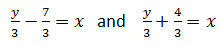A (4, 3) B (−4, 3) C (6, 2) D (5, −2) E The lines do not intersect
Question 3 Explanation:
The correct answer is (E). Because both of these equations are already solved for the variable x, we can set them equal to each other to find the value of y. Begin by multiplying both sides by 3 to remove the denominator.

y − 7 = y + 4

Notice that this equation will never be true. Since there is no solution, so we can conclude that the lines do not intersect.
 Question 4
An integer, x, is multiplied by 2. This value is subtracted from 5 and the quantity is squared. This result is equivalent to one fourth of x summed with x times itself 3 times. Which of the following equations represents this equality?

 A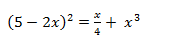B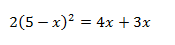C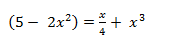D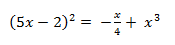E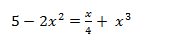Question 4 Explanation:
The correct answer is (A). Translate the description into a mathematical expression. 2x is subtracted from 5 and squared:
(5 – 2x)2 which is equal to (¼)(x) + 3x.
(5 − 2x)2 = x4 + x3
 Question 5
The slope of the line represented by the equation 2(x − 3) − 6y = 10 is equal to what?

 A −½ B 1/3 C ½ D ¼ E 2
Question 5 Explanation:
The correct answer is (B). Recall the slope intercept form of a line:
y = mx + b where m is the slope.

Solve the given equation for y to find the slope:

2x − 6 − 6y = 10
−6y = −2x + 16
y = 1/3x16/6

Slope is equal to 1/3.
 Question 6
If the side length of a square is z units, what is the length of its diagonal in terms of z?

 A z − 1 B z C z21⁄2 D z² E z³
Question 6 Explanation:
The correct answer is (C). Recall that the diagonal of a square cuts it into 2 right triangles with side length ratios of x, x, x√2. With side length of z, the diagonal will be z212
 Question 7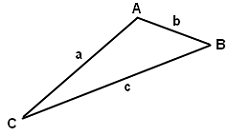The triangle ABC that is provided has side lengths of a, b. and c feet and is not a right triangle. Let A’ be the image when the triangle is reflected across side BC. Which of the following is an expression for the perimeter, in feet, of quadrilateral A’BAC?

 A 2ab B 2(a + b) - c C a + b + 2c D 2(b + c) E 2(a + b)
Question 7 Explanation:
The correct answer is (E). One approach this question is to draw it.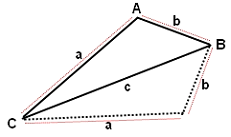For the resulting parallelogram A'BAC, length c will not be included in the perimeter (since CB is the diagonal of the parallelogram, not a side).

All the answer choices with length c can be eliminated -- which leaves us with just (A) and (E).

The perimeter can be found by adding up the lengths of the sides:
a + a + b + b
= 2a + 2b
= 2(a + b)
 Question 8
If 60% of a number is 12, what is 165% of the same number?

 A 20 B 22 C 28 D 30 E 33
Question 8 Explanation:
The correct answer is (E). Translate the given expressions into equations:
x * 0.6 = 12
x = 20
20 * 1.65 = 33
 Question 9
The ratio of circle A’s circumference to circle B’s circumference is 2:9, what is the ratio of their areas?

 A 1:32 B 1:22 C 1:20.25 D 2:23 E 2:25
Question 9 Explanation:
Let the circumference of the smaller circle be c = 2πr
Let the circumference of the larger circle be C = 2πR
We are told that the ratio of circle A’s circumference to circle B’s circumference is 2:9
Thus: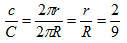The area of circle A with radius ‘r’ is πr²
The area of circle B with radius ‘R’ is πR²
The ratio of the areas of the two circles is: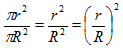So, the ratio of the areas of the two circles is equal to the square of the ratio of the radii of the two circles. We can substitute the ratio of the radii obtained above into the formula for the ratio of the areas:

The ratio of the areas of the two circles is:Question 10
Which coordinates satisfy the inequality: y + 3 > −3(x − 2)

 A (−1, −2) B (0, 2) C (1, 0) D (−3, 15) E (2, −2)
Question 10 Explanation:
The correct answer is (D). Solve the inequality for one variable:
y + 3 > −3x + 6
y > −3x + 3

This states that the y-coordinate must be larger than −3 times the x-coordinate plus 3. Test the points provided to see which one satisfies the given inequality (this can also be done graphically). Only (−3, 15) satisfies the inequality.
Once you are finished, click the button below. Any items you have not completed will be marked incorrect.
There are 10 questions to complete.
 ← List →